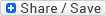Front page Sunday, June 21, 2009 Why are most genetic associations found through candidate gene studies wrong?   posted by p-ter @ 6/21/2009 03:47:00 PMIn a recent post, I made a blanket statement that the vast majority of candidate gene association studies published in psychiatric genetics (actually, in nearly all fields of genetics) are wrong. I'm not just being offhandedly dismissive--below, I outline the statistical argument behind that claim. This discussion is cribbed almost verbatim from a discussion of the issue by statisticians at the Welcome Trust. Let's assume that there are a finite number of loci in genome, and we test some number of those (in a genome-wide association study, this is on the order of 500K-1M; in a candidate gene study it's more likely in the tens. But the actual marker density is irrelevant for what follows) for association with some phenotype of interest. In general, the criterion used to decide if one has discovered a true association is the p-value, or the probability of seeing the data that you have given that there is no association. But that's not really the quantity you're interested in. The real quantity of interest is the probability that there's a true association given the data you see--the inverse of what's being reported. By Bayes' Law, this probability depends on the prior probability of an association at that marker, the p-value threshold you've chosen to call a finding "significant", and crucially, the power you had to detect the association . Thus, the interpretation of a given p-value depends on the power to detect an association, such that the lower your power, the lower the probability that a "significant" association is true . That's where recent evidence from large genome-wide association studies comes into play. For nearly all diseases, reproducible associations have small effect size and are only detectable when one has sample sizes in the thousands or tens of thousands (for many psychiatric phenotypes, even studies with these sample sizes don't seem to find much). The vast majority of candidate gene association studies had sample sizes in the low hundreds, and thus had essentially zero power to detect the true associations. By the argument above, in this situation the probability that a "significant" association is real approaches zero. The problem with candidate gene association studies is not that they were only targeting candidate genes, per se, but rather that they tended to have small sample sizes and were woefully underpowered to detect true associations.  Let D be the data, T be the event that an association is true, t, be the event that an association is not true, and P(T) be the prior probability that an association is true. P(T|D) = P(D|T)P(T) / [ P(D|T) P(T) + P(D|t) (1-P(T) ]P(D|T) is the power, and P(D|t) is the p-value. Clearly, both are relevant here.  http://jnci.oxfordjournals.org/cgi/content/full/96/6/434#FD1 As the authors note, A key point from both perspectives is that interpreting the strength of evidence in an association study depends on the likely number of true associations, and the power to detect them which, in turn, depends on effect sizes and sample size. In a less-well-powered study it would be necessary to adopt more stringent thresholds to control the false-positive rate. Thus, when comparing two studies for a particular disease, with a hit with the same MAF and P value for association, the likelihood that this is a true positive will in general be greater for the study that is better powered, typically the larger study. In practice, smaller studies often employ less stringent P-value thresholds, which is precisely the opposite of what should occur.Labels: Genetics input{width:180px; border: thin #000000 inset;} .sub{width:180px; border: thin #000000 inset;}Razib's Home Page GNXP Archives Interviews Blogroll